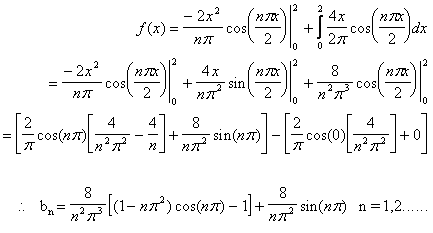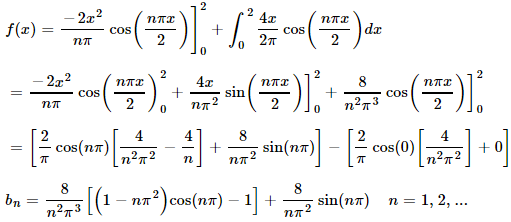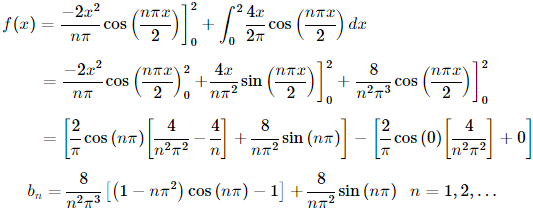Search IntMath
Close

# Comparison of math Web publishing options

By Murray Bourne, 01 Dec 2014

## Comparison of ASCIIMathML, PHPMathPublisher, MathJax, KaTeX, MathTeX and images for math publishing

Updated: Nov 2017

The following chart compares various math Web publishing options. Any of these can be used in blogs and forums (with some setup), and some can be used for sending math in an email.

## Note

1. "Ease of setup" refers to what the Webmaster needs to do.
2. "Ease of use" refers to how easy it is for math publishers and students to use
3. The green marker indicates a "pass" for that criterion.

## 1. Math using ImagesMath rendered using an image. Note the TImes New Roman font.
Description Traditional Web development - create images on local computer, include in HTML file, upload the lot to server Create images and HTML, upload everything to the server. Fiddly - need to create images locally and then upload to server Yes (because the math is in images) Yes (but slow) Yes (because the math is in images) I used to use images for all math on IntMath, but no longer, so I don't have an example to point to!

## 2 ASCIIMathML

Description One script resides on server. User inputs (simple and easy) code. Browser processes code and displays math using text. Very easy and light - just needs one JS file on your server (or you can just insert a link) Very easy to use - entering math is like calculator input (and you can use HTML symbols). Realistic to get students to use this solution. Only works on Firefox (or IE with a plugin). Rendering in other browsers is poor, because it gives MathML output. But when used with MathJax (see below), ASCIIMathML is a good cross-browser solution. Slow to render - doesn't render properly (this is similar to non-Firefox desktop browsers) No (Relatively slow on mobile, and may not render well.) Syntax for entering math using ASCIIMathML ASCIIMathML input, MathJax output

## 3. PHP Math Publisher (no longer under development)

(No longer available.)

Description Scripts, fonts and images reside on server. User inputs LaTeX into HTML file. Browser processes code and displays math as images. Easy set up on server (just a few files). Caches images after creating them. Fiddly - requires LaTeX input Yes (because images) Yes (because images) Yes (because images)

## 4. MathJaxScreen shot of math rendered using MathJax
Description Scripts, fonts and images reside on server. User inputs LaTeX into HTML file. Browser processes code and displays math using text and images. Allows for "copy-paste math", where you can copy equations from (say) Wikipedia. Now that MathJax allows ASCIIMathML input, it's very easy for students to use. Easy - just call the MathJax script at the top of the page Can input math using MathML or LaTeX. Yes Yes, but slow No MathJax – render math on the Web on all browsers (Best in Chrome, Firefox, Safari. Relatively fast on mobile, but KaTeX is faster.) Easy math input and nice output using ASCIIMathML and MathJax Using MathJax fonts outside of MathJax

## 5. KaTeXScreen shot of math rendered using KaTEX
Description Similar approach to MathJax, in that scripts, fonts and images reside on server. User inputs LaTeX into HTML file. Browser processes code and displays math using text and images. Much faster than MathJax, as it's simpler and doesn't try to do as much. Is still under development, so does not provide all the functionality of MathJax (yet). For example, it doesn't display matrices, split functions and many math symbols. Easy - just call the KaTeX script at the top of the page Requires LaTeX input, so not so easy for students to cope with. (But it can be made to work with ASCIIMathML.) Yes (but some small issues where older mobile browsers don't play well) Yes, quicker than MathJax No Equivalent Fractions (desktop version) Most of the desktop version of IntMath now uses KaTeX. KaTeX - a new way to display math on the Web KaTeX and MathJax Comparison Demo KaTeX with ASCIIMathML input and MathJax fallback

## 6. MathTeX

Description LaTeX processor on server. User inputs source code for image in LaTeX format. Image is created by server, on the fly. Very troublesome to set up LaTeX with mathtex-cgi on server. Fiddly - requires LaTeX input. Yes (because images) Yes (because images) Yes (because images) MathTex home page (Last time I looked, the equations were not loading on that page. This is quite old software now.) (Any browser. Relatively fast on mobile.)

## 7. jqMath

Description jqMath follows a similar approach to MathJax and KaTeX. That is, it renders TeX in the browser using javascript. jqMath uses a simple TeX-like syntax, with MathML semantics. In my view, it would benefit from using standard LaTeX fonts (as MathJax and KaTeX do). Easy - just call the jqMath script at the top of the page Requires TeX input, so not so easy for students to cope with. Yes (but some small issues where older mobile browsers don't play well) Yes, quicker than MathJax No jqMath (I don't use jqMath anywhere on IntMath.)

## Conclusion

While there are some very good attempts at solving the problem of getting math onto a Web page in the above applications, none of them really solves all the following requirements:

1. Easy to install
2. Easy to use (especially for students)
3. Cross-browser
4. Mobile-friendly (small file sizes, low CPU requirements)

MathTeX needs an easy to use WYSIWYG editor. While there are various LaTeX editors, they all require a 2-step process (create the code in one application, then copy it to somewhere else for the Web).

Using KaTeX with ASCIIMathML input is the best solution so far (and that's what I am using throughout IntMath now).

I hope this comparison has been of some use to you.

### Comment Preview

HTML: You can use simple tags like <b>, <a href="...">, etc.

To enter math, you can can either:

1. Use simple calculator-like input in the following format (surround your math in backticks, or qq on tablet or phone):
a^2 = sqrt(b^2 + c^2)
(See more on ASCIIMath syntax); or
2. Use simple LaTeX in the following format. Surround your math with $$ and $$.
$$\int g dx = \sqrt{\frac{a}{b}}$$
(This is standard simple LaTeX.)

NOTE: You can mix both types of math entry in your comment.